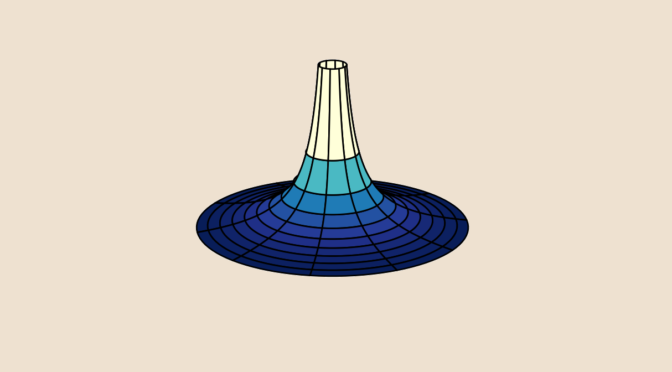Can you paint a surface with infinite area with a finite quantity of paint? For sure… let’s do it!

Consider the 3D surface given in cylindrical coordinates as $S(\rho,\varphi):\begin{cases} x &= \rho \cos \varphi\\ y &= \rho \sin \varphi\\ z &= \frac{1}{\rho}\end{cases}$ for $$(\rho,\varphi) \in [1,\infty) \times [0, 2 \pi)$$. The surface is named Gabriel’s horn.

### Volume of Gabriel’s horn

The volume of Gabriel’s horn is $V = \pi \int_1^\infty \left( \frac{1}{\rho^2} \right) \ d\rho = \pi$ which is finite.

### Area of Gabriel’s horn

The area of Gabriel’s horn for $$(\rho,\varphi) \in [1,a) \times [0, 2 \pi)$$ with $$a > 1$$ is: $A = 2 \pi \int_1^a \frac{1}{\rho} \sqrt{1+\left( -\frac{1}{\rho^2} \right)^2} \ d\rho \ge 2 \pi \int_1^a \frac{d \rho}{\rho} = 2 \pi \log a.$ As the right hand side of inequality above diverges to $$\infty$$ as $$a \to \infty$$, we can conclude that the area of Gabriel’s horn is infinite.

### Conclusion

Gabriel’s horn could be filled with a finite quantity of paint… therefore painting a surface with infinite area. Unfortunately the thickness of the paint coat is converging to $$0$$ as $$z$$ goes to $$\infty$$, leading to a paint which won’t be too visible!